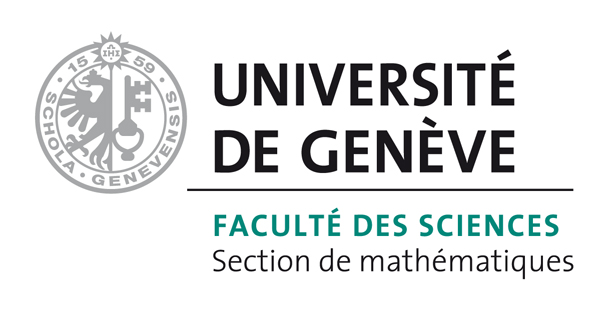# Bart Vandereycken## A globally convergent method to compute the real stability radius for time-delay systems

by , , ,

#### Abstract:

This paper presents a novel algorithm to compute the real stability radius for a linear delay system of retarded type with multiple delays. The real stability radius is the distance to instability, measured as the minimal real-valued perturbation that renders the system unstable. Our method is based on characterizing this distance to instability as the inverse of the global maximum of a real structured singular value function. We develop a criss-cross type algorithm that globally converges to this maximum, and whose convergence rate seems to be superlinear and sometimes quadratic in numerical experiments. The algorithm exploits that the intersections of these singular value functions with constant functions can be written as purely imaginary eigenvalues of certain delay eigenvalue problems (DEP) with positive and negative delays. This is an extension of the well-known linear case (without delays) where this results in algebraic eigenvalue problems. In addition, a novel numerical solver to compute all the imaginary eigenvalues of this DEP is also presented. It combines an approximation using spectral discretizations and an automatic procedure to determine the required number of discretization points. Finally, due to the presence of multiple eigenvalues at the maximum, these approximations are corrected with a block-Newton algorithm for nonlinear eigenvalue problems.

#### Reference:

F. Borgioli, W. Michiels, D. Lu, B. Vandereycken, "A globally convergent method to compute the real stability radius for time-delay systems", In Systems & Control Letters, vol. 127, pp. 44-51, 2019.

#### Bibtex Entry:

@article{Borgioli_MLV:2019,
Abstract = {This paper presents a novel algorithm to compute the real stability radius for a linear delay system of retarded type with multiple delays. The real stability radius is the distance to instability, measured as the minimal real-valued perturbation that renders the system unstable. Our method is based on characterizing this distance to instability as the inverse of the global maximum of a real structured singular value function. We develop a criss-cross type algorithm that globally converges to this maximum, and whose convergence rate seems to be superlinear and sometimes quadratic in numerical experiments. The algorithm exploits that the intersections of these singular value functions with constant functions can be written as purely imaginary eigenvalues of certain delay eigenvalue problems (DEP) with positive and negative delays. This is an extension of the well-known linear case (without delays) where this results in algebraic eigenvalue problems. In addition, a novel numerical solver to compute all the imaginary eigenvalues of this DEP is also presented. It combines an approximation using spectral discretizations and an automatic procedure to determine the required number of discretization points. Finally, due to the presence of multiple eigenvalues at the maximum, these approximations are corrected with a block-Newton algorithm for nonlinear eigenvalue problems.},
Author = {Borgioli, F. and Michiels, W. and Lu, D. and Vandereycken, B.},
Journal = {Systems {\&} Control Letters},
Title = {A globally convergent method to compute the real stability radius for time-delay systems},
Year = {2019},
Pages = {44--51},
Volume = {127},
Pdf = {http://www.unige.ch/math/vandereycken/papers/published_Borgioli_MLV_2019.pdf},
Doi = {10.1016/j.sysconle.2019.03.009}
}